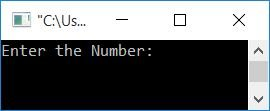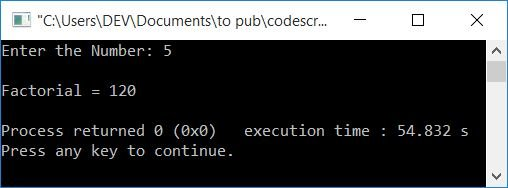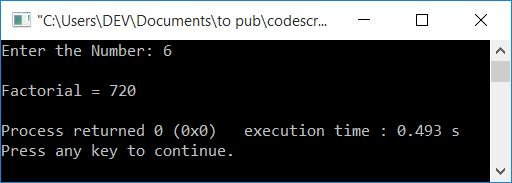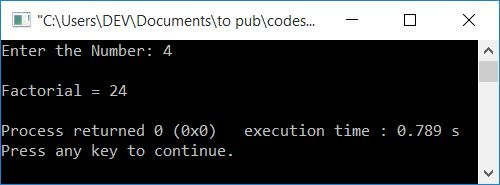# C++ program to find the factorial of a number

In this article, you will learn and get code to find and print the factorial of a number entered by the user at run-time in C++. The factorial program is created in the following ways:

### How to Determine Factorial

To find the factorial of a number n, here is the formula:

`factorial = n*(n-1)*(n-2)*...*3*2*1`

For example, the factorial of a number, say 6, is:

```6! = 6*5*4*3*2*1
= 30*12*2
= 30*24
= 720```

Note: The symbol ! indicates factorial.

## Find the factorial of a number in C++ using the for loop

To find the factorial of a number in C++ programming, you have to ask the user to enter the number. Then find and print the factorial of that number using the formula given above.

This program finds and prints the factorial of a number using a for loop. The question is: write a program in C++ that finds the factorial of a given number using a for loop. Here is its answer:

```#include<iostream>
using namespace std;
int main()
{
int num, i, fact=1;
cout<<"Enter the Number: ";
cin>>num;
for(i=num; i>=1; i--)
fact = fact*i;
cout<<"\nFactorial = "<<fact;
cout<<endl;
return 0;
}```

This program was built and runs under the Code::Blocks IDE. Here is its sample run:Now supply any number, say 5, and press the ENTER key to find and print its factorial as shown in the snapshot given below:The following is the dry run of the above program with user input 5:

• fact=1 at the start.
• When the user enters 5 as input, then it gets stored in num. So num=5
• Now the evaluation of the for loop gets started after receiving the number from the user.
• That is, the value of num (5) gets initialized to i. So i=5. The initialization part (first part) of the for loop executes first, but only once.
• Now that the condition i>=1 or 5>=1 has been evaluated as true, program flow enters the loop, and fact*i or 1*5 or 5 is initialized to fact
• The program flow goes to the update part (third part) of the for loop, which decrements the value of i. So i=4
• The condition i>=1 is evaluated once more.
• Every time after updating the value of i, the condition gets evaluated.
• And each time, the program flow enters the loop after evaluating the condition, until the condition is determined to be false.
• Therefore, before its condition is evaluated to be false, here is the value of i and the fact after each evaluation:
• i=5, fact=5
• i=4, fact=20
• i=3, fact=60
• i=2, fact=120
• i=1, fact=120
• Now, after exiting from the loop, just print the value of fact; that will be the factorial result of the given number.

### In C++, using the while loop, find the factorial of a number

Here is another program to find the factorial of a number, but using the while loop in C++. In the while loop, there is only one part, which is for condition. So we have to put the initialization part before the loop and update parts inside its body.

```#include<iostream>
using namespace std;
int main()
{
int num, i, fact=1;
cout<<"Enter the Number: ";
cin>>num;
i=num;
while(i>=1)
{
fact = fact*i;
i--;
}
cout<<"\nFactorial = "<<fact;
cout<<endl;
return 0;
}```

This program produces the same output as the previous program.

### Using a user-defined function to find the factorial of a number in C++

Now let's create the same-purpose program using a user-defined function named findFact(). This function receives a number as its argument and returns the factorial of the number.

```#include<iostream>
using namespace std;
int findFact(int);
int main()
{
int num, fact;
cout<<"Enter the Number: ";
cin>>num;
fact = findFact(num);
cout<<"\nFactorial = "<<fact;
cout<<endl;
return 0;
}
int findFact(int n)
{
int i, f=1;
for(i=n; i>=1; i--)
f = f*i;
return f;
}```

Here is its sample run with user input, 6:### Using call-by-reference to find the factorial of a number in C++

Now this is the end of this article. This program also calculates factorial, but using the call-by-reference method. That is, the address of the variable fact gets passed as an argument to the findFact() function. So any change to this variable directly affects the original value.

```#include<iostream>
using namespace std;
void findFact(int, int *);
int main()
{
int num, fact=1;
cout<<"Enter the Number: ";
cin>>num;
findFact(num, &fact);
cout<<"\nFactorial = "<<fact;
cout<<endl;
return 0;
}
void findFact(int n, int *f)
{
int i;
for(i=n; i>=1; i--)
*f = (*f)*i;
}```

Here is its sample run with user input, 4:#### The same program in different languages

C++ Quiz

« Previous Program Next Program »